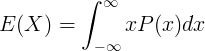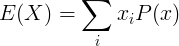# Expectation (E)

## Expectation Value

In probability and statistics, the expectation or expected value, is the weighted average value of a random variable.

### Expectation of continuous random variableE(X) is the expectation value of the continuous random variable X

x is the value of the continuous random variable X

P(x) is the probability density function

### Expectation of discrete random variableE(X) is the expectation value of the continuous random variable X

x is the value of the continuous random variable X

P(x) is the probability mass function of X

### Properties of expectation

##### Linearity

When a is constant and X,Y are random variables:

E(aX) = aE(X)

E(X+Y) = E(X) + E(Y)

##### Constant

When c is constant:

E(c) = c

##### Product

When X and Y are independent random variables:

E(X ⋅Y) = E(X) ⋅ E(Y)

### conditional expectation

Currently, we have around 929 calculators and conversion tables to help you "do the math" quickly in areas such as:

And we are still developing more. Our goal is to become the one-stop, go-to site for people who need to make quick calculations or who need to find quick answer for basic conversions.

Additionally, we believe the internet should be a source of free information. Therefore, all of our tools and services are completely free, with no registration required. We coded and developed each calculator individually and put each one through strict, comprehensive testing. However, please inform us if you notice even the slightest error – your input is extremely valuable to us. While most calculators on Justfreetools.com are designed to be universally applicable for worldwide usage, some are for specific countries only.